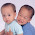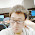## Permutation Sequence

The set [1,2,3,…,n] contains a total of n! unique permutations.
By listing and labeling all of the permutations in order,
We get the following sequence (ie, for n = 3):
1. "123"
2. "132"
3. "213"
4. "231"
5. "312"
6. "321"
Given n and k, return the kth permutation sequence.
Note: Given n will be between 1 and 9 inclusive.

### Analysis:

One way to solve the problem (can only pass the small test) is to generate from the 1st permutation to the required one (similar to the problem Next permutation.). However, when n=9, the last permutation is the 362880th one, which is too time consuming.

A better way is to fully use the properties of permutation: the total number of permutation with length n is n!.
First, let's take a look at how permutation works:
Major steps are swap and fix:
Here in order to grow the tree,  every time start the first unfixed element in each node, generate child nodes by swapping the first element with every other element.The leave nodes are those do not have element to swap.

So, when we have the idea of how to generate the permutation, next step is to generate it faster. Think the tree in this way:

ABC
/                   |                    \
ABC             BAC              CBA
/       \             /      \               /    \
ABC  ACB     BAC BCA    CBA CAB

Every leave node is a permutation. Take a look at the second level, each subtree (second level nodes as the root), there are (n-1)! permutations in it.  Totally there are n nodes in 2nd level, thus the total number of permutations are n*(n-1)!=n!.

So, what we want to do is to locate one permutation among the leave nodes. The simple method is to generate and search each leave node until we find the one. Now, what we can do here we want to skip some trees to reduce the complexity. e.g. in the above tree, if we know the required leave node is in the third sub tree (under CBA), we can skip the first two and search from the "CBA".

Say we have the required permutation is the kth one, first we can locate which subtree it belongs to in the 2nd level, by computing s = k / ((n-1)!). Then we get the sth subtree, and set k=k%((n-1)!) , now search the sub tree until we get the leave node. How to get the sth subtree root node? Just like the idea of how permutation works (the first figure): Just put the sth elment after fixed letter.  e.g. ABCD,  we want the 3rd subtree root node in 2nd level, just put C in the 1st place, which is CABD;   For ABCDE, we want the 3rd subtree root node in the 3rd level, it is ADBCE.

### Code(C++, loop):

class Solution {
public:
string getPermutation(int n, int k) {
// Start typing your C/C++ solution below
// DO NOT write int main() function
int nf={1,2,6,24,120,720,5040,40320,362880}; //n factorail
string res;//result string
for (int i=1;i<=n;i++){res+=char(i+'0');} // initialization
k--;
for (int i=0;i<n;i++){
int m = k%nf[n-i-1];
int s = k/nf[n-i-1];
if (m==0 && s==0){ return res;}
else{
if (s>0){
for (int j=i-1+s; j>i-1;j--){
char tmp = res[j];
res[j]=res[j-1];
res[j-1]=tmp;
}
if (m==0){return res;}
}
k=m;
}
}
return res;
}
};


### Code(c++, recursion):

class Solution {
public:
void swap(string &str,int st, int l){
for (int i=st+l;i>st;--i){
char tmp = str[i];
str[i]=str[i-1];
str[i-1]=tmp;
}
}

void getP(string &str,int &st, int &n,int &k, int *nf){
if (k==0 || st>n-1){
return;
}else{
swap(str,st,k/nf[n-st-1]);
k=k%nf[n-st-1];
getP(str,++st,n,k,nf);
}
}

string getPermutation(int n, int k) {
string str="";
int nf={1,1,2,6,24,120,720,5040,40320,362880}; //n factorail
for (int i=1;i<=n;i++){str+=char(i+'0');}
int st=0;
getP(str,st,n,--k,nf);
return str;
}
};

### Code (Python, Recursion):

class Solution:
def getP(self,strs,st,n,k,nf):
if k==0 or st>n-1:
return
else:
l=k/nf[n-st-1]
p=strs.pop(st+l)
strs.insert(st,p)
k=k%nf[n-st-1]
self.getP(strs,st+1,n,k,nf)
return

# @return a string
def getPermutation(self, n, k):
strs = [str(x) for x in range(1,n+1)]
nf=[1,1,2,6,24,120,720,5040,40320,362880]
st=0
k=k-1
self.getP(strs,st,n,k,nf)
return "".join(x for x in strs)



1.According to your analysis, the 5th element should be "321", not "312".

1.Oh, thanks for commenting, the figure is actually from the web (not draw by myself), which is only a illustration of the general idea. You can find the details from the code, which also output the correct answer. Thanks.

2.I have checked the recursion version of the permutation, the program actually runs slightly different from what we analyze (the idea of the tree is the same), because we do "swap()" then "perm()" and the recursion "swap()" step is the reason why the figure shows different from the actually running result. In my opinion, the backtracking "swap()" swaps the current version of number, instead of the root number (e.g. when it goes to 231, then backtracking ,swap to 213, then backtracking again swap to 312). If I do not explain it clear, please take a look at the recursion version of the code. Hope this reply can help you. Thanks. :)

2.Thanks for answering, this definitely helps. You have some best c++ solution for LeetCode.

3.class Solution:
def permute(self, num):
n=len(num)
tot=[]
if n==1:
return [num]
elif n==2:
return [num,[num,num]]
else:
for x in self.permute(num[0:n-1]):
for i in range(n):
y=x[0:i]+[num[n-1]]+x[i:n-1]
tot.append(y)

4.Although the loop version is pass the OJ, it has a flaw,

according to your int nf={1,2,6,24,120,720,5040,40320,362880}; when i = 0, nf = 120 which is wrong,
and also the j = i - 1 + s doesn't make sense, if you have nf = {1, 1,2,6,24,120,720,5040,40320,362880}
the for loop change to for(int j = i + s; j > i; j--) make much sense. The modified version also pass the leetcode oj. Thank you.

5.first we can locate which subtree it belongs to in the 2nd level, by computing s = k / ((n-1)!). why is it k/(n-1)!?

6.This comment has been removed by the author.

7.The clustermap for your website is interesting!

8.Thanks for the explanation!!